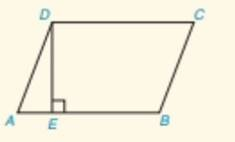Chapter 4.CT, Problem 3CT### Elementary Geometry for College St...

6th Edition
Daniel C. Alexander + 1 other
ISBN: 9781285195698

#### Solutions

Chapter
Section### Elementary Geometry for College St...

6th Edition
Daniel C. Alexander + 1 other
ISBN: 9781285195698
Textbook Problem
1 views

# In ▱ ABCD, AD=5 and DC=9. If the altitude from vertex D to A B ¯ has length 4(that is, DE=4), find the length of E B ¯ .                                     _ .To determine

To Find:

Length of EB.

Explanation

Given:

Given parallelogram is ABCD,

AD = 5 and DC = 9.

Calculation:

From the figure,

DE¯AE¯, and DEA is a right angle triangle and hence ΔDEA is right triangle with a hypotenuse DA¯.

From the Pythagorean Theorem,

DA2=DE2+AE2

### Still sussing out bartleby?

Check out a sample textbook solution.

See a sample solution

#### The Solution to Your Study Problems

Bartleby provides explanations to thousands of textbook problems written by our experts, many with advanced degrees!

Get Started

#### In Exercises 1124, find the indicated limits, if they exist. 11. limx0 (5x 3)

Applied Calculus for the Managerial, Life, and Social Sciences: A Brief Approach

#### Solve the equations in Exercises 126. 2x3x2x3x+1=0

Finite Mathematics and Applied Calculus (MindTap Course List)

#### Solve the equation for x. 46. |2x1x+1|=3

Single Variable Calculus: Early Transcendentals, Volume I

#### Rewritten as an iterated integral in polar coordinates,

Study Guide for Stewart's Multivariable Calculus, 8th

#### For f(x) = tanh1 2x, f(x) = a) 2(sech1 2x)2 b) 2(sech2 2x)1 c) 214x2 d) 114x2

Study Guide for Stewart's Single Variable Calculus: Early Transcendentals, 8th

#### Find each value. logaa

College Algebra (MindTap Course List)

#### Explain the distinction between a hypothesis and a prediction.

Research Methods for the Behavioral Sciences (MindTap Course List)